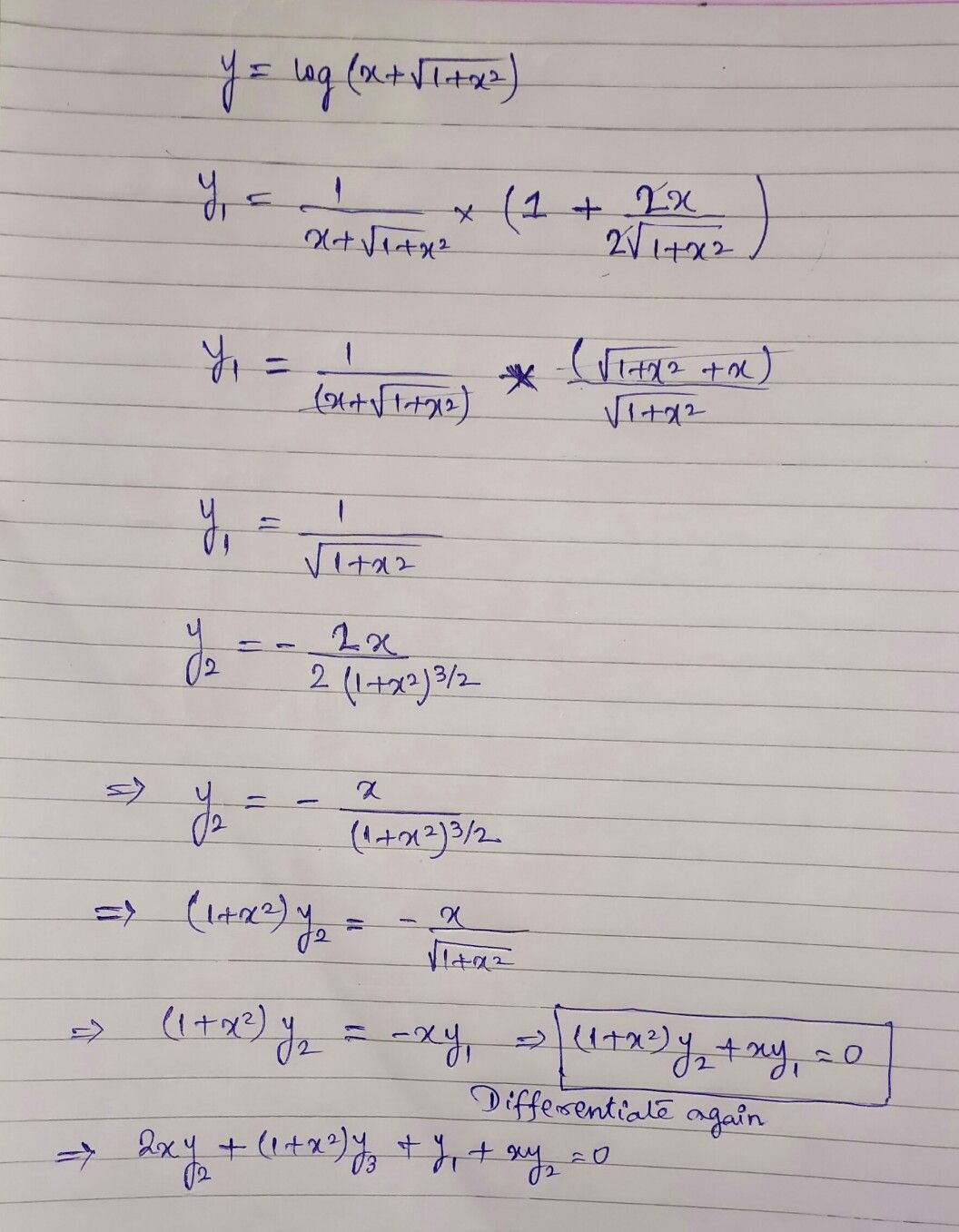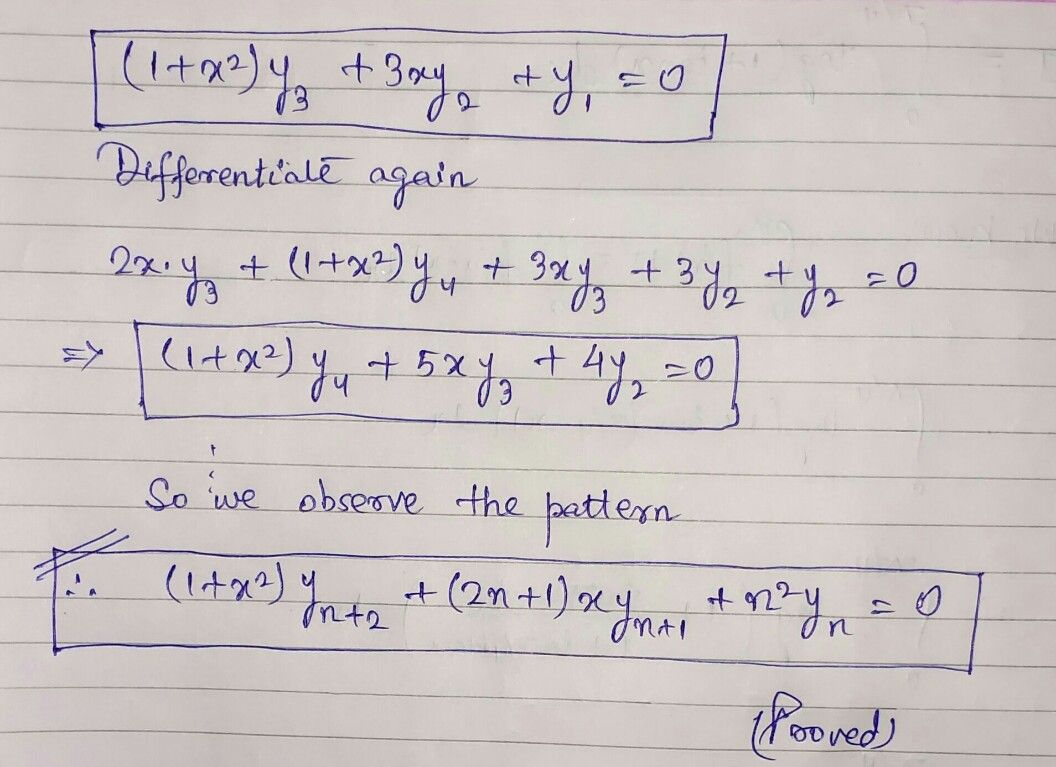Symbol
Problem$r$ $2.$ If $y=log\left(x+\sqrt{1+x^{2}} \right)$ then prove that $\left(1+x^{2}\right)y_{n+2}+\left(2n+1\right)$ $xy_{n+1}+n^{2}y_{n}=0$
Calculus
Search count: 105
SolutionQanda teacher - KanekiIf any doubt kindly ask will clarify